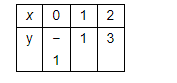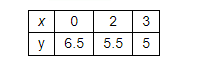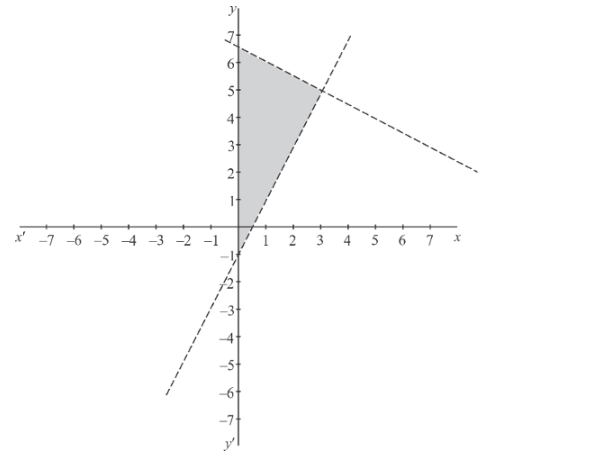# Draw the graphs of following equations:`
Question:

Draw the graphs of following equations:

2x − = 1 and x + 2y = 13

(i) find the solution of the equations from the graph.

(ii) shade the triangular region formed by the lines and the y-axis.

Solution:

Here we have to draw the graph between two equations given by…… (1)…… (2)

Also we have to find the solution of the given equations.

The first equation can written as follow…… (3)

Now we are going to find the value of y at different value of xNow mark the points (0,-1), (1,1) and (2,3) on xy-plane and we will draw a line which pass through these points.

The second equation can be written as

$y=\frac{13-x}{2}$....(4)Now mark the points (0, 6.5), (2, 5.5) and (3, 5) on xy-plane and we will draw a line which pass through these points.

From the above analysis we have the following graphForm the above graph, the intersection point of the two lines is the solution.

$x=3$

$y=5$

And also the triangular region is shaded in the figure.